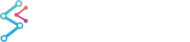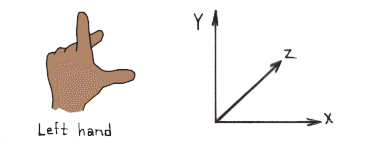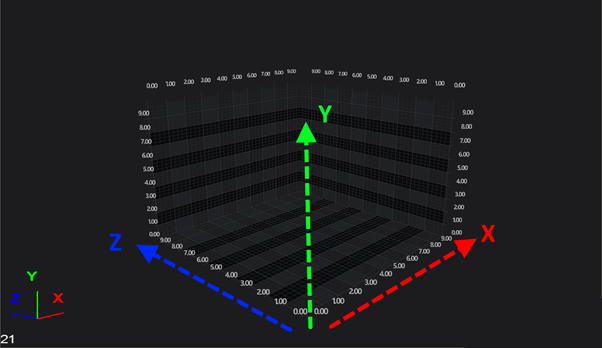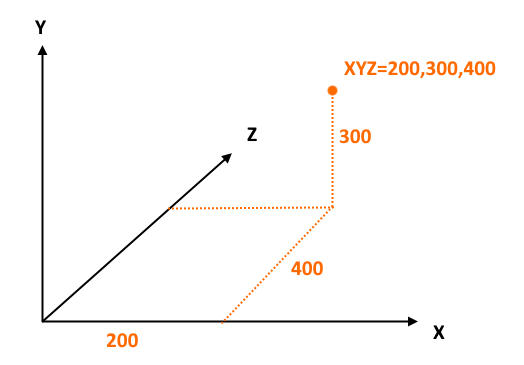﻿ Left Handed vs. Right Handed Coordinate Systems | WPF Chart DocumentationLeft Handed vs. Right Handed Coordinate Systems

## The Left Handed Coordinate System (LHS)

The SciChart3DSurface by default renders a 3D world using the Left Handed Coordinate system or LHS (as is common to DirectX). In the LHS X and Z form the horizontal plane, and Y is always up YDirection=(0,1,0). It is helpful to think of the 3D world as a 2D Chart in X-Y and Z goes ‘into the screen’.Left handed coordinate system. X-Z is a horizontal plane, Y is up.#### World Coordinates

Objects in 3D space are measured in World Coordinates, which are floating point Vector3's. For instance, an object with a position of X,Y,Z = 200,300,400 is located at this virtual ‘World Coordinate’ in the 3D world. In the Right Handed Coordinate system (RHS), which is the default in SciChart3D, this object will be positioned as follows.NOTE: 3D coordinates do not correspond to screen coordinates, although it is possible to convert between the two (with some limitations).

#### Data Coordinates

By contrast, the data you pass to SciChart3D is measured in Data Coordinates. This data-space can be any data-type (double, float, int, DateTime, TimeSpan) and must be transformed to World Coordinates before the object is displayed.

The transformation is performed by the Axis. For example, NumericAxis3DLogarithmicNumericAxis3DDateTimeAxis3D or TimeSpanAxis3D.

### Conversion between Data and World Coordinates

The SciChart AxisCore type contains conversion functions to convert between data & world coordinates.

This API is the same as the API presented for 2D Charts Converting Data to Pixel Coordinates. Except, in the case of a 3D Axis, the pixel coordinate is a World coordinate.